Next: Circulation and Vorticity Up: Incompressible Inviscid Flow Previous: Flow over a Broad-Crested

# Vortex Lines, Vortex Tubes, and Vortex Filaments

The curl of the velocity field of a fluid, which is generally termed vorticity, is usually represented by the symbol, so that(4.71)

A vortex line is a line whose tangent is everywhere parallel to the local vorticity vector. The vortex lines drawn through each point of a closed curve constitute the surface of a vortex tube. Finally, a vortex filament is a vortex tube whose cross-section is of infinitesimal dimensions.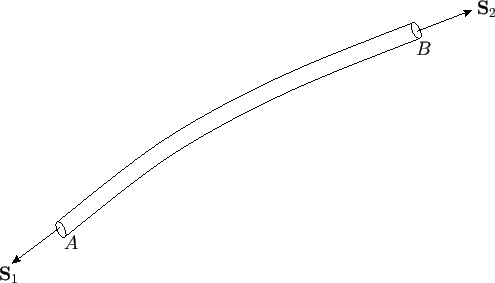Consider a section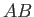of a vortex filament. The filament is bounded by the curved surface that forms the filament wall, as well as two plane surfaces, whose vector areas are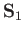and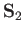(say), which form the ends of the section at pointsand, respectively. (See Figure 4.9.) Let the plane surfaces have outward pointing normals that are parallel (or anti-parallel) to the vorticity vectors,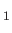and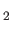, at pointsand, respectively. The divergence theorem (see Section A.20), applied to the section, yields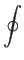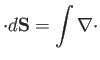(4.72)

where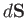is an outward directed surface element, anda volume element. However,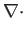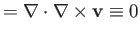(4.73)

[see Equation (A.173)], implying that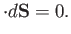(4.74)

Now,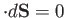on the curved surface of the filament, becauseis, by definition, tangential to this surface. Thus, the only contributions to the surface integral come from the plane areasand. It follows that(4.75)

This result is essentially an equation of continuity for vortex filaments. It implies that the product of the magnitude of the vorticity and the cross-sectional area, which is termed the vortex intensity, is constant along the filament. It follows that a vortex filament cannot terminate in the interior of the fluid. For, if it did, the cross-sectional area,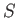, would have to vanish, and, therefore, the vorticity,, would have to become infinite. Thus, a vortex filament must either form a closed vortex ring, or must terminate at the fluid boundary.

Because a vortex tube can be regarded as a bundle of vortex filaments whose net intensity is the sum of the intensities of the constituent filaments, we conclude that the intensity of a vortex tube remains constant along the tube.Next: Circulation and Vorticity Up: Incompressible Inviscid Flow Previous: Flow over a Broad-Crested
Richard Fitzpatrick 2016-03-31